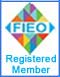Note: No part of this website may be reproduced, stored in a retrieval, or transmitted in any form or by any mean

## Our ProductsHome » Nano Science Instruments   »   Thermal Conductivity Apparatus Thermal Conductivity Apparatus Objective Thermal Conductivity of Liquids & Nanofluids & Engine Oils etc.Thermal Conductivity Apparatus Code: TCA-01 Thermal Conductivity ApparatusTheory The Apparatus follows widely accepted theory of heat conduction in liquids based on Debyes concept in which the hydroacoustic vibrations (phonons) of a continuous medium(base fluid) are responsible for the heat transfer in liquids. Based on this heat transfer mechanism, Bridgman has obtained a formula, characterized by the direct proportionality between thermal conductivity and sound velocity in pure liquids.where νs = ultrasound velocity, N (Avogadro's number) = 6.02×1023 and V (molar volume) =KB= (Boltzmann's constant) = 1.3807×10-23 J/K For Polyatomic Liquids it was modified as:For Nanofluids it was further modified by J.Hemalatha as under:where, kbm is the thermal conductivity value obtained through the modified Bridgman equation, ρnf is the density of nanofluid, andis the molar mass of nanofluid. xbf and xp are the molar fractions of the base fluid and nanoparticle respectivelywhereas Mbf and Mp are the respective molar masses of the base fluid and nanoparticle.Working PrincipleUltrasound waves of known frequency are produced and its wavelength is measured. Then sound velocity in LiquidAfter calculating velocity of sound in Liquid, one can calculate the thermal conductivity by the formula given by P W Bridgman. Description Thermal Conductivity Apparatus consists of following parts: Electronic Unit,Conductivity Cell-2MHz,Stability Cell 4MHz to increase settling time of the suspension,Temperature Controller Unit-To maintain temp.of liquids / nanofluids at desired temp from RT to 70°C.